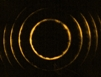Interferometric Optics

# GENERALIZED QUANTUM INTERFERENCE

## Since 1987

These are references on the optics derived from the application of Dirac's bra-ket notation to N-slit interferometry. As explained in the references, Dirac's quantum approach is applicable to the propagation of a single photon or to the propagation of ensembles of indistinguishable photons.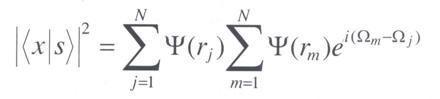The nexus between the quantum probability interference equation and the measurable intensity is described and explained in Fundamentals of Quantum Entanglement, 2nd Edn (Institute of Physics, Bristol, 2022) where the difference between the physics of quantum interference equations and the physics of classical interference, is also explained. This book also the generalized N-slit interference equations in 2 dimensions and 3 dimensions and provides in detail the probability amplitude equations describing:

• Hambury Brown-Twist Interferometers.
• HOM Interferometers.
• Mach-Zehnder Interferometers.
• Michelson Interferometers.
• Sagnac Interferometers

The following terminology, and concepts, are discussed in the given references:

# Extremely expanded laser beam illumination (up to 3000:1)

## Synonym: Extremely elongated Gaussian beam illumination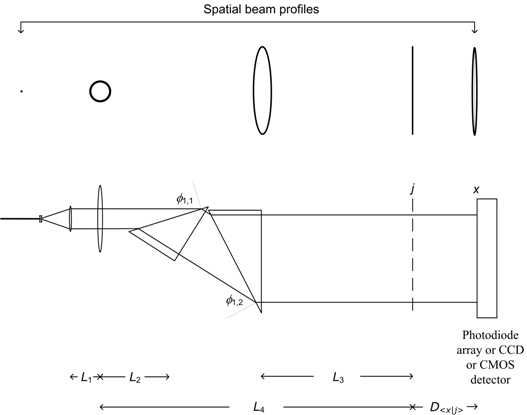Optical architecture for the multiple-prism beam expander microscope/nanoscope (MPBEM/N). The beam incident on the object can be, for example, 25-60 mm wide X 20 µm high. This is an extremely elongated beam (in the plane of propagation) with a width to height ratio in the range of 1000:1 to 3000:1. This type of illumination has also become known as light sheet illumination. Comparisons between theory and experiments, in the NSLI configuration, have been performed for even values (N = 2, 4, 6...) and odd values (N = 3, 5, 7...) of N. This includes the cases of two-slit interference, three-slit interference, four-slit interference, etc. (Duarte, 1991, 2002, 2005). For reviews see Tunable Laser Optics and Tunable Laser Applications).

The 1991 and 1993 papers also reported, for the first time, on the use of quantum mechanics techniques, via Dirac's notation, in the field of imaging. In addition, these papers illustrated the prediction of measured interferograms using interferometric equations derived using Dirac's quantum notation.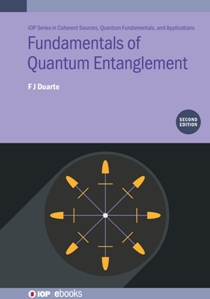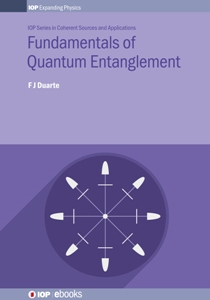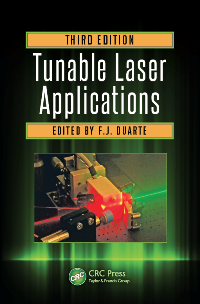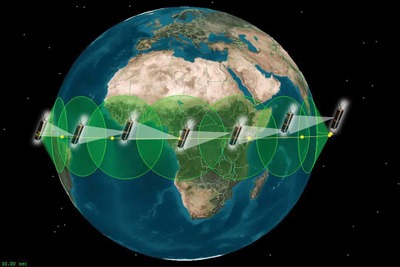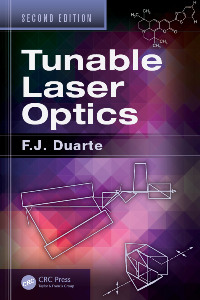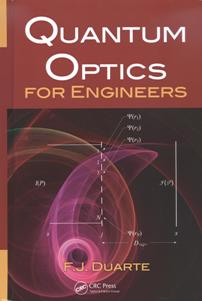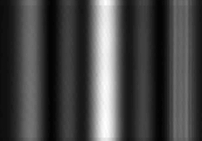*Derives the cavity linewidth equation using Dirac's notation to describe the intracavity propagation in a dispersive laser oscillator.

**Introduced the concept of N-slit interferometric microscopy. Also demonstrated, for the first time, the use of quantum mechanics techniques in the field of imaging and the use of extremely elongated thin beams (of approximate dimensions 25-50 mm wide X 25µm high) as illumination sources in microscopy and microdensitometry. This type of one-dimensionally beam-expanded illumination for microscopy has also become known as thin light sheet microscopy (TLSM) and selective plane illumination microscopy (SPIM).

***This note explains the Dirac approach to interference, in the context of the laser, and is included in the editor's choice for papers published in the American Journal of Physics for the 1988-2001 period (R. H. Romer, Editor's choice, Am. J. Phys. 69, 635-647 (2001)).

****Introduced the concept of interferometric characters in secure optical communications.

*****This note expands slightly on the 1997 paper and explains how Dirac's quantum notation is used to provide a unified description of interference, diffraction, refraction, and reflection. It also describes why the probabilistic single-photon generalized interference equation, derived using Dirac's notation, can be applied to the description of interference of large numbers of indistinguishable photons, or ensemble of indistinguishable photons, observed in the macroscopic domain.

## DIRAC AND INTERFERENCE

The first description of interference directly applicable to the interference of narrow-linewidth high-power laser beams was prophetically given by Dirac in 1930 . Dirac's description of interference was not always understood, or accepted, and is referred to by some as "Dirac's dictum." Dirac's description of interference was explained, using practical laser terminology:

"... interference can be analyzed via the interaction of probability amplitudes. These probability amplitudes are said to be represented by wave functions. Hence, interference can be described via the multiplication of an addition of complex wave functions, with its corresponding complex conjugate. Dirac writes about a [monochromatic] beam of light consisting of a large number of photons... In the case of a large number of indistinguishable photons his words are just fine" .

"The Dirac discussion... begins with reference to a beam of roughly monochromatic light; then prior to his dictum on interference, he writes about a beam of light having a large number of photons... In present terms this is no different than the description of interference due to the interaction of a high-power narrow-linewidth laser beam with a two-beam interferometer" 

In the previous description a key concept is that a beam of monochromatic light is a beam is indistinguishable photons which is equivalent to a beam of narrow-linewidth laser emission .

References

1. F. J. Duarte, Tunable Laser Optics (Elsevier-Academic, New York, 2003) Chapter 2.

2. F. J. Duarte, Interference of two independent sources, Am. J. Phys. 66, 662-663 (1998).

## PATENTS BASED ON DIRAC OPTICS

Keywords: double-slit interference, double-slit interferometer, double-slit interferometry, four-slit interference, four-slit interferometer, four-slit interferometry, N-slit interference, N-slit interferometer, N-slit interferometry, quantum, quantum imaging, two-slit interference, two-slit interferometer, two-slit interferometry, three-slit interference, three-slit interferometer, three-slit interferometry, triple-slit interference, triple-slit interferometer, triple-slit interferometry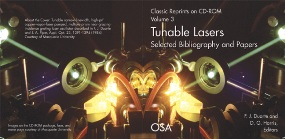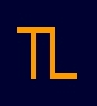Page published on the 9th of July, 1997. Updated on the 17th of May, 2023.